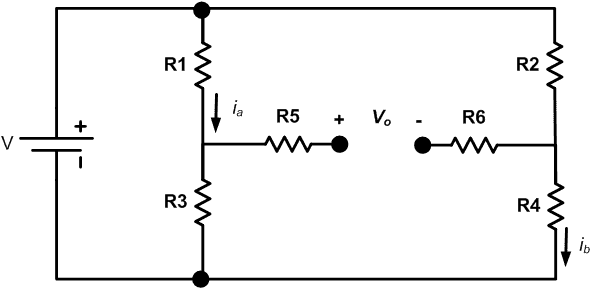# Determine ia and Vo

## Homework StatementDetermine ia and Vo

V=0.4 V, R1 = 7kΩ, R2 = 28 kΩ, R3 = 43 kΩ, R4 = 31 kΩ

V= i*R

## The Attempt at a Solution

Does R5 and R 6 cancel out since the pair of parallel resistors share a common node, making Vo - 0 V?

lewando
Homework Helper
Gold Member
Does R5 and R 6 cancel out since the pair of parallel resistors share a common node, making Vo - 0 V?
They don't "cancel out" (weak terminology). There is no current going through them so no voltage drop across them. Better to say that their values are not relevant to the solution of the problem. Vo is not 0V. You have 2 voltage dividers-- evaluate the midpoint voltage of both of them.

Last edited:
Thank you.